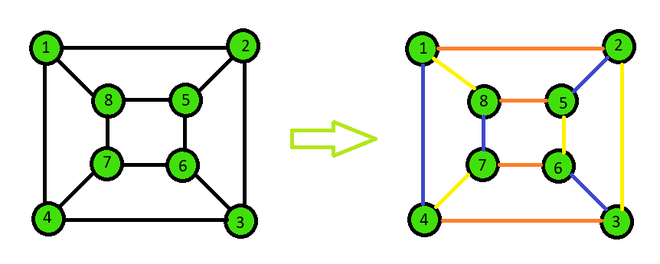Related Articles
Java Program to Find Chromatic Index of Cyclic Graphs
• Last Updated : 05 Feb, 2021

Chromatic Index of a graph is the minimum number of colours required to colour the edges of the graph such that any two edges that share the same vertex have different colours.

Whereas, the cyclic graph is a graph that contains at least one graph cycle i.e. cyclic means a path from at least one node back to itself. Here given, the cyclic graph we have to find the chromatic index of that graph.

Examples:

Input: e = 12

edges = {{ 1, 2}, { 2, 3}, { 3, 4},

{ 4, 1}, { 5, 6}, { 6, 7},

{ 7, 8}, { 8, 5}, { 1, 8},

{ 2, 5}, { 3, 6}, { 4, 7}}

Output: Chromatic Index = 3

Explanation:Approach:

By applying Vizing’s Theorem we can prove that a given graph can have a chromatic index of ‘d’ or ‘d’+1, where d is the maximum degree of the graph.

Below is the step-by-step approach of the algorithm:-

1. Initialize the number of edges and the edge list.
2. Color the graph according to the Vizing’s Theorem.
3. Assign a color to an edge and check if any adjacent edges have the same color or not.
4. If any adjacent edge has the same color, then increment the color to try the next color for that edge.
5. Repeat till all the edges get it’s color according to the theorem.
6. Once done print the maximum value of color for all the edges and the colors of every edge.

Below is the implementation of the above approach:

## Java

 `// Java program to find the chromatic``// index of a cyclic graph``import` `java.util.*;`` ` `public` `class` `chromaticIndex {`` ` `    ``// Function to find the chromatic index``    ``public` `void` `edgeColoring(``int``[][] edges, ``int` `e)``    ``{``        ``// Initialize edge to first``        ``// edge and color to color 1``        ``int` `i = ``0``, color = ``1``;`` ` `        ``// Repeat until all edges are done coloring``        ``while` `(i < e) {``            ``// Give the selected edge a color``            ``edges[i][``2``] = color;``            ``boolean` `flag = ``false``;``            ``// Iterate through all others edges to check``            ``for` `(``int` `j = ``0``; j < e; j++) {``                ``// Ignore if same edge``                ``if` `(j == i)``                    ``continue``;``                ``// Check if one vertex is similar``                ``if` `((edges[i][``0``] == edges[j][``0``])``                    ``|| (edges[i][``1``] == edges[j][``0``])``                    ``|| (edges[i][``0``] == edges[j][``1``])``                    ``|| (edges[i][``1``] == edges[j][``1``])) {``                    ``// Check if color is similar``                    ``if` `(edges[i][``2``] == edges[j][``2``]) {``                        ``// Increment the color by 1``                        ``color++;``                        ``flag = ``true``;``                        ``break``;``                    ``}``                ``}``            ``}`` ` `            ``// If same color faced then repeat again``            ``if` `(flag == ``true``) {``                ``continue``;``            ``}`` ` `            ``// Or else proceed to a new vertex with color 1``            ``color = ``1``;``            ``i++;``        ``}`` ` `        ``// Check the maximum color from all the edge colors``        ``int` `maxColor = -``1``;``        ``for` `(i = ``0``; i < e; i++) {``            ``maxColor = Math.max(maxColor, edges[i][``2``]);``        ``}`` ` `        ``// Print the chromatic index``        ``System.out.println(``"Chromatic Index = "` `+ maxColor);``    ``}`` ` `    ``// Driver code``    ``public` `static` `void` `main(String[] args)``    ``{`` ` `        ``// Number of edges``        ``int` `e = ``4``;`` ` `        ``// Edge list``        ``int``[][] edges = ``new` `int``[e][``3``];`` ` `        ``// Initialize all edge colors to 0``        ``for` `(``int` `i = ``0``; i < e; i++) {``            ``edges[i][``2``] = -``1``;``        ``}`` ` `        ``// Edges``        ``edges[``0``][``0``] = ``1``;``        ``edges[``0``][``1``] = ``2``;`` ` `        ``edges[``1``][``0``] = ``2``;``        ``edges[``1``][``1``] = ``3``;`` ` `        ``edges[``2``][``0``] = ``3``;``        ``edges[``2``][``1``] = ``4``;`` ` `        ``edges[``3``][``0``] = ``4``;``        ``edges[``3``][``1``] = ``1``;`` ` `        ``// Run the function``        ``chromaticIndex c = ``new` `chromaticIndex();``        ``c.edgeColoring(edges, e);``    ``}``}`
Output
```Chromatic Index = 2
```

Output:

`Chromatic Index = 2`

References:

Attention reader! Don’t stop learning now. Get hold of all the important Java Foundation and Collections concepts with the Fundamentals of Java and Java Collections Course at a student-friendly price and become industry ready. To complete your preparation from learning a language to DS Algo and many more,  please refer Complete Interview Preparation Course.

My Personal Notes arrow_drop_up